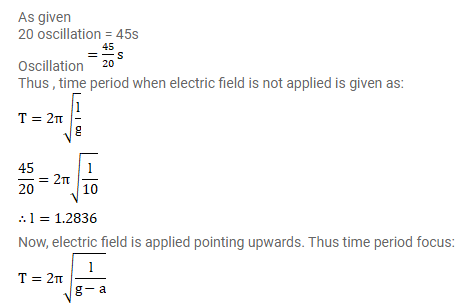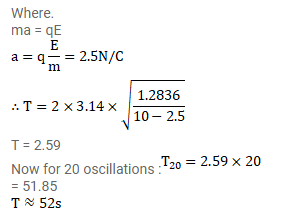# The bob of a simple pendulum has a mass ofQuestion:

The bob of a simple pendulum has a mass of $40 \mathrm{~g}$ and a positive charge of $4.0 \times 10^{-6} \mathrm{C}$. It makes 20 oscillations in $45 \mathrm{~s}$. A vertical electric field pointing upward and of magnitude $2.5^{\times 10^{4}} \mathrm{~N} / \mathrm{C}$ is switched on. How much time will it now take to complete 20 oscillations?

Solution: